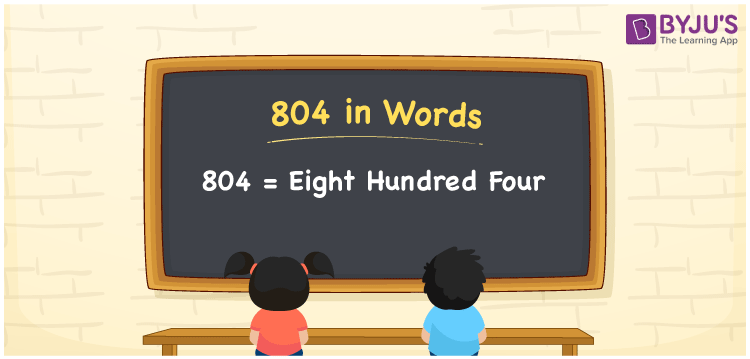# 804 in Words

804 in Words is “Eight hundred four”. The number 804 has three digits and its ones, tens and hundreds place helps to write the number name. It is also a cardinal number. For example, the cost of 7 books is Rs. 804. This article will guide you to write the number 804 in words using the place value system in detail.

 804 in Words: Eight Hundred Four. Eight Hundred Four in Numerical Form: 804.

## 804 in English Words## How to Write 804 in Words?

Learn the place values of 804 using the below table:

 Hundreds Tens Ones 8 0 4

The expanded form of 804 is as follows:

= 8 × Hundred + 0 × Ten + 4 × One

= 8 × 100 + 0 × 10 + 4 × 1

= 800 + 4

= 804

= eight hundred four

Hence, 804 in words is eight hundred four.

804 in words – Eight hundred four

Is 804 an odd number? – No

Is 804 an even number? – Yes

Is 804 a perfect square number? – No

Is 804 a perfect cube number? – No

Is 804 a prime number? – No

Is 804 a composite number? – Yes

## Frequently Asked Questions on 804 in Words

Q1

### How to spell 804?

804 in words is eight hundred four.

Q2

### Simplify 800 + 4, and express it in words.

Simplifying 800 + 4, we get 804. Hence, 804 in words is eight hundred four.

Q3

### Is 804 an even number?

Yes, 804 is an even number.